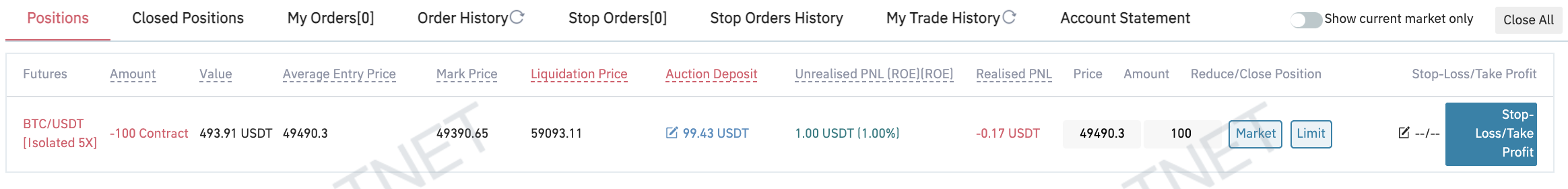• From- To time of the change range
Theme
Bright
Classic black
Pitch black
• Language
• 简体中文
• English
• Tiếng Việt
• 繁体中文
• Español
• Русский язык
• Français
• Deutsch
• Italiano
• Português
• ภาษาไทย
• Indonesia
• Türkçe
• 日本語
Exchange Rate Switch
• CNY
• USD
• KRW
• VND
• EUR
• GBP
• HKD
• JPY
• RUB
• TRY
• INR

### Gate.io Help Center

Customer Support / TicketGate.io > Help Center > Futures > Tutorial of Intermediate Contract Trading
##### More article in the group
How to Calculate Return on Investment (ROI)##### Gate.io
Updated at:56 days 16 hours ago
lv

Return on Investment (ROI) refers to a ratio that compares the PNL of a position from a contract investment relative to its cost, in other words, a ratio that suggests an investment's profitability. ROI is one of the key metrics in evaluating the potential return from an investment.

ROI produces an approximate measure of the profitability of a given contract investment based on the calculated results, which can help investors evaluate their investment, learn more about their profits and losses, and optimize asset allocation.

### ROI Calculation Formula:

ROI = Unrealized PNL/Margin*100%

Note: In Cross Margin Mode, the margin is the entire available balance in the trader's contract account. But when calculating ROI, use the minimum margin displayed on the webpage. Use the actual margin in the calculation when in Isolated Margin Mode.

### Example:As the screenshot above shows, the unrealized PNL of this position is 1USDT and the margin is 99.43USDT. Therefore, the ROI of this position is: ROI = Unrealized PNL/Margin*100% ROI =1/99.43*100% ROI ≈ 1%

Can't find the answer you want?Submit a Ticket

Popular Articles

Articles